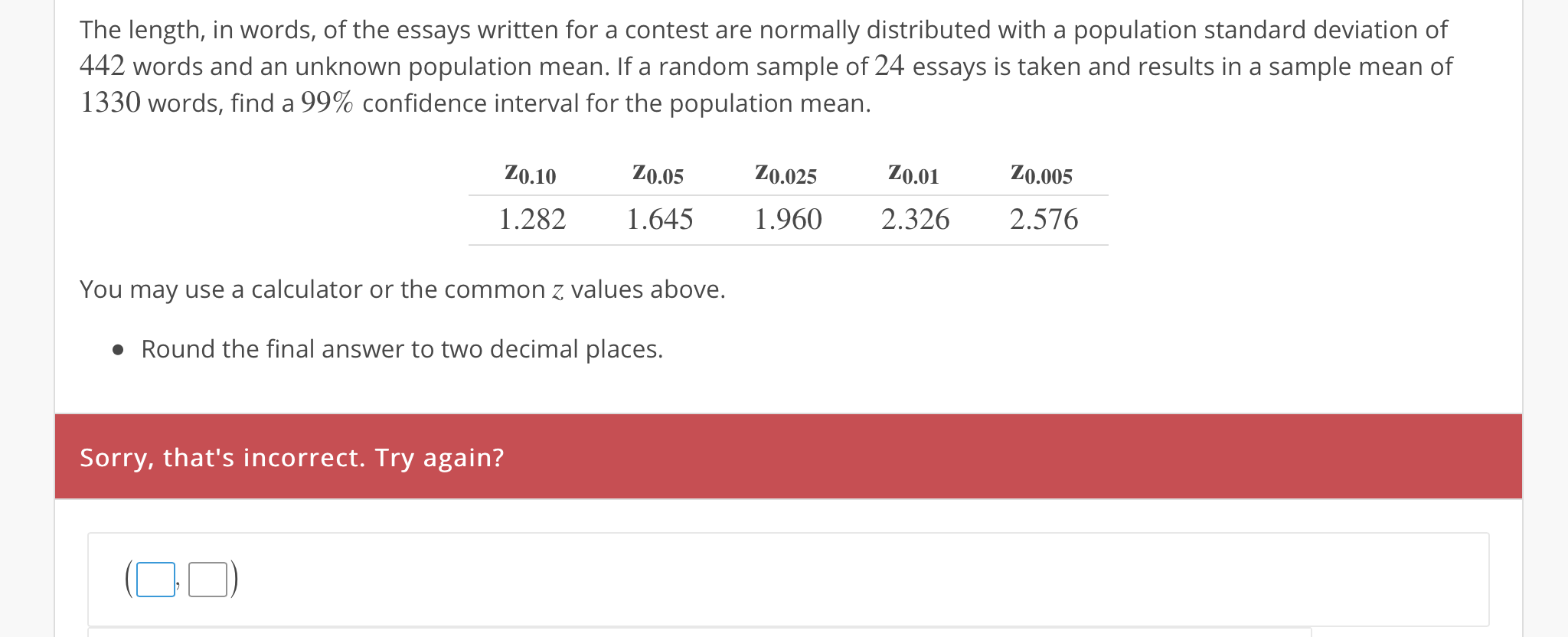# The length, in words, of the essays written for a contest are normally distributed with a population standard deviation of442 words and an unknown population mean. If a random sample of 24 essays is taken and results in a sample mean of1330 words, find a 99% confidence interval for the population mean.Z0.10Z0.05Z0.025 Z0.0 Z0.0051.282 .645 .960 2.326 2.576You may use a calculator or the common z values above.Round the final answer to two decimal places,Sorry, that's incorrect. Try again?0

Questionhelp_outlineImage TranscriptioncloseThe length, in words, of the essays written for a contest are normally distributed with a population standard deviation of 442 words and an unknown population mean. If a random sample of 24 essays is taken and results in a sample mean of 1330 words, find a 99% confidence interval for the population mean. Z0.10Z0.05Z0.025 Z0.0 Z0.005 1.282 .645 .960 2.326 2.576 You may use a calculator or the common z values above. Round the final answer to two decimal places, Sorry, that's incorrect. Try again? 0 fullscreen
check_circle

Step 1

Confidence Interval:

The form of the confidence interval is,

CI = Point estimate ± Margin of error

The approximate 100*(1–α)% confidence interval for the population mean will be obtained using the formula given below:

Step 2

Obtain the critical value:

The required confidence level is 100*(1–α)% = 99%.

The required conditions for using standard normal distribution to estimate the confidence interval about mean is given below:

• The population standard deviation σ must be known.
• Either the sample size (n) must be greater than 30 or the population must be normally distributed.

Here, it is given that the population of lengths in words of the essays written for a contest is normally distributed and the population standard deviation is known.

From the given common z-values using standard normal distribution the critical value is obtained as 2.576 from the calculation given below:

Step 3

Find the 99% confidence interval for the population mean length of words:

Given that, the population standard deviation is σ = 442,

The sample mean is x-bar = 1,330,

The sample size is n = 24,

The critical value is z(α/2) = z0.005 = ...

### Want to see the full answer?

See Solution

#### Want to see this answer and more?

Solutions are written by subject experts who are available 24/7. Questions are typically answered within 1 hour.*

See Solution
*Response times may vary by subject and question.
Tagged in

### Other